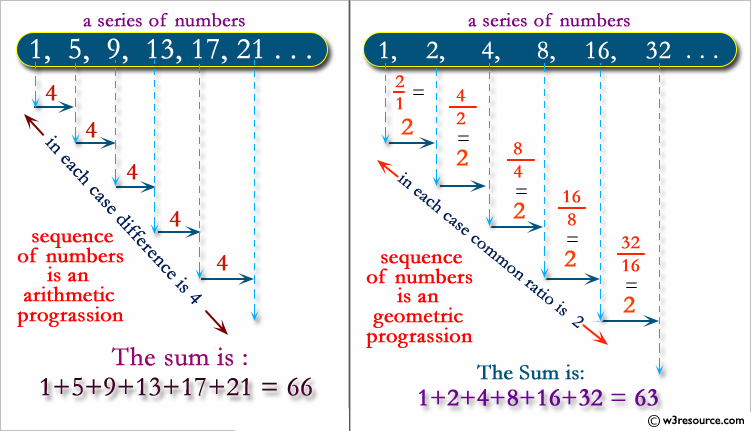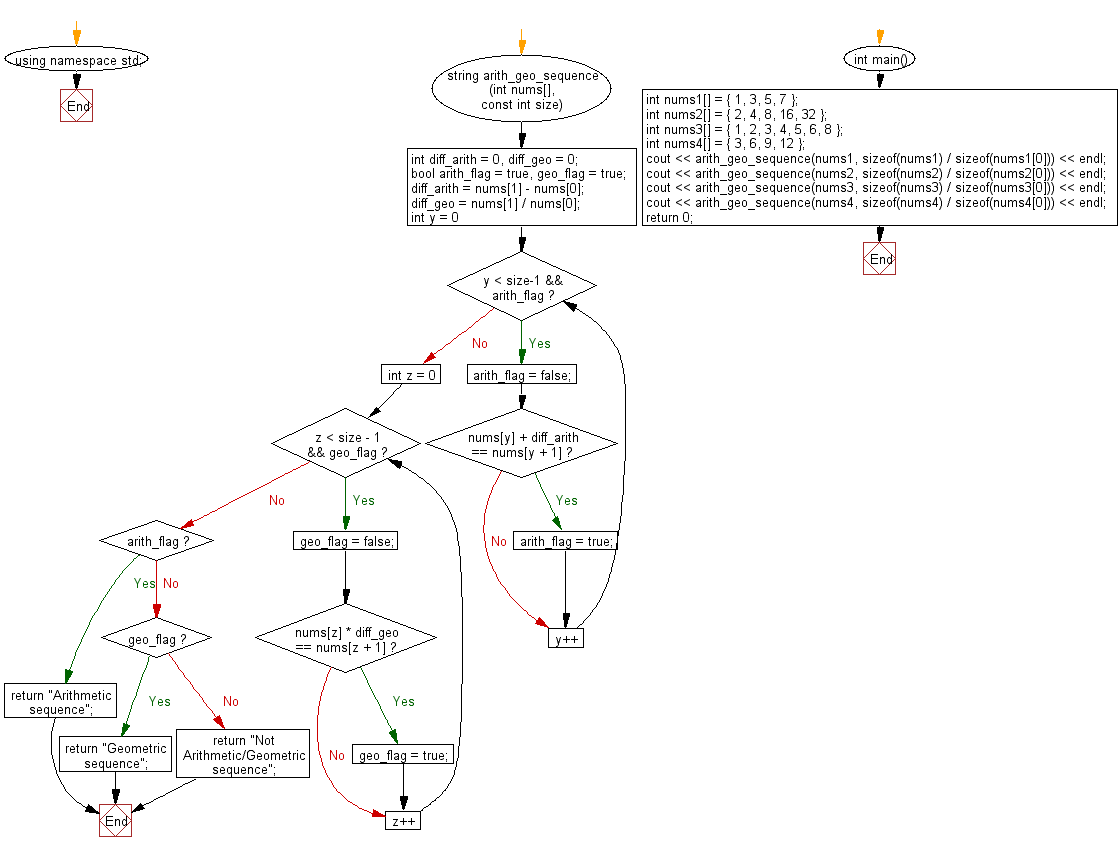﻿ C++ : Check if the sequence of the numbers is in a array

# C++ Exercises: Check whether the sequence of the numbers in a given array is a “Arithmetic” or “Geometric” sequence

## C++ Basic: Exercise-84 with Solution

Write a C++ program to check whether the sequence of the numbers in a given array is an "Arithmetic" or "Geometric" sequence. Return -1 if the sequence is not "Arithmetic" or "Geometric".

From Wikipedia
In mathematics, an arithmetic progression (AP) or arithmetic sequence is a sequence of numbers such that the difference between the consecutive terms is constant. Difference here means the second minus the first. For instance, the sequence 5, 7, 9, 11, 13, 15, . . . is an arithmetic progression with common difference of 2.
In mathematics, a geometric progression, also known as a geometric sequence, is a sequence of numbers where each term after the first is found by multiplying the previous one by a fixed, non-zero number called the common ratio. For example, the sequence 2, 6, 18, 54, ... is a geometric progression with common ratio 3. Similarly 10, 5, 2.5, 1.25, ... is a geometric sequence with common ratio 1/2.

Pictorial Presentation:Sample Solution:

C++ Code :

``````#include <iostream>
#include <string>

using namespace std;

string arith_geo_sequence(int nums[], const int size) {

int diff_arith = 0, diff_geo = 0;
bool arith_flag = true, geo_flag = true;

diff_arith = nums - nums;
diff_geo = nums / nums;

for (int y = 0; y < size-1 && arith_flag; y++)
{
arith_flag = false;

if (nums[y] + diff_arith == nums[y + 1])
{
arith_flag = true;
}
}
for (int z = 0; z < size - 1 && geo_flag; z++)
{
geo_flag = false;

if (nums[z] * diff_geo == nums[z + 1])
{
geo_flag = true;
}
}

if (arith_flag)
{
return "Arithmetic sequence";
}
else if (geo_flag)
{
return "Geometric sequence";
}
else
{
return "Not Arithmetic/Geometric sequence";
}
}

int main() {

int nums1[] = { 1, 3, 5, 7 };
int nums2[] = { 2, 4, 8, 16, 32 };
int nums3[] = { 1, 2, 3, 4, 5, 6, 8 };
int nums4[] = { 3, 6, 9, 12 };
cout << arith_geo_sequence(nums1, sizeof(nums1) / sizeof(nums1)) << endl;
cout << arith_geo_sequence(nums2, sizeof(nums2) / sizeof(nums2)) << endl;
cout << arith_geo_sequence(nums3, sizeof(nums3) / sizeof(nums3)) << endl;
cout << arith_geo_sequence(nums4, sizeof(nums4) / sizeof(nums4)) << endl;
return 0;
}
``````

Sample Output:

```Arithmetic sequence
Geometric sequence
Not Arithmetic/Geometric sequence
Arithmetic sequence
```

Flowchart:C++ Code Editor:

What is the difficulty level of this exercise?

﻿

## C++ Programming: Tips of the Day

Why is there no std::stou?

The most pat answer would be that the C library has no corresponding "strtou", and the C++11 string functions are all just thinly veiled wrappers around the C library functions: The std::sto* functions mirror strto*, and the std::to_string functions use sprintf.

Ref: https://bit.ly/3wtz2qA

We are closing our Disqus commenting system for some maintenanace issues. You may write to us at reach[at]yahoo[dot]com or visit us at Facebook